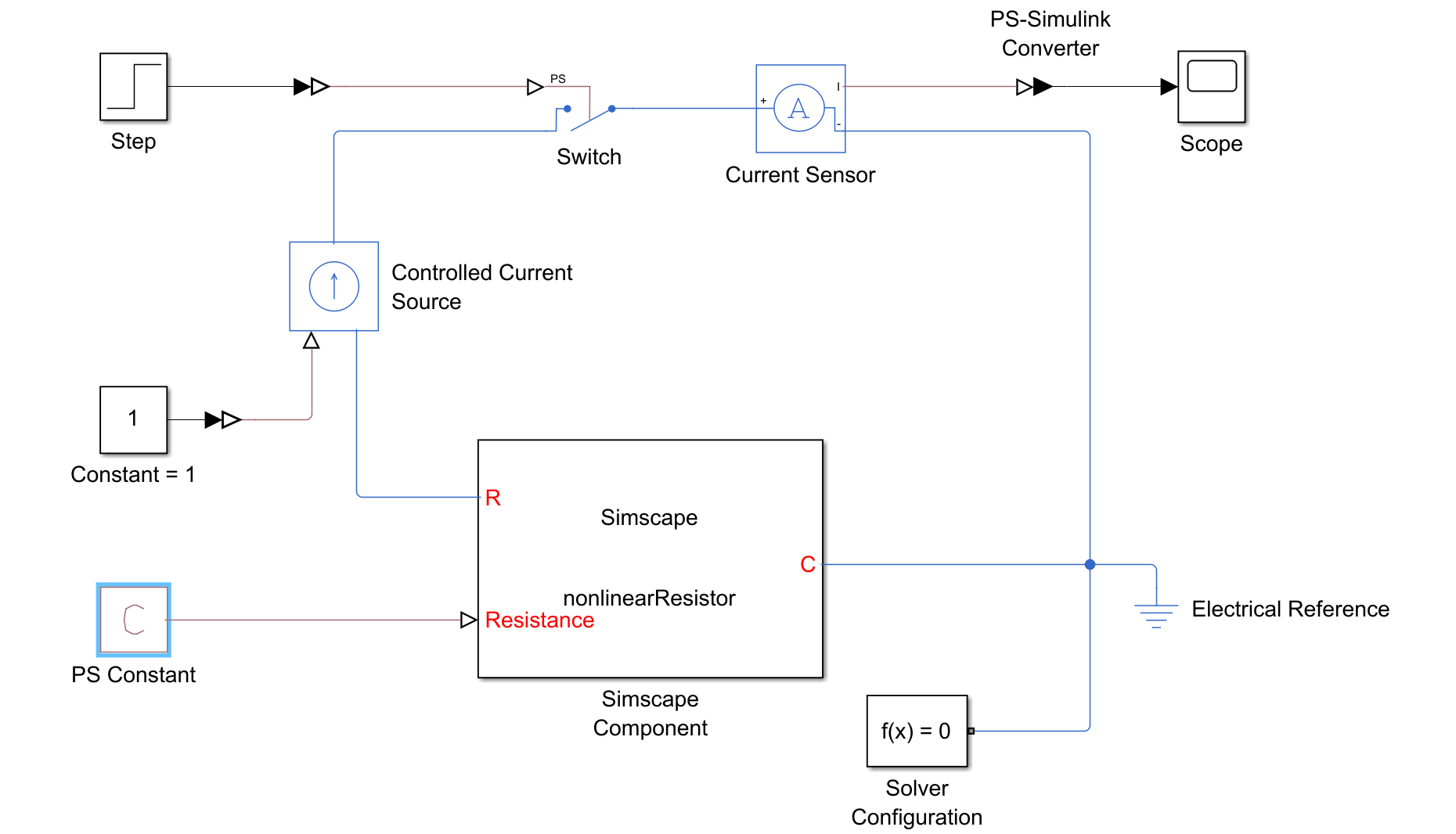## Resolving Issues with Nonlinearities

Nonlinearities add computational complexity, which slows down simulation. Partitions that contain nonlinearities with respect to owned states are incompatible with HDL Coder™. To use HDL Coder with networks that use the partitioning solver, you must resolve these partitions. To check whether a model contains nonlinearities with respect to owned states, open the Statistics Viewer tool and update the results. Under the Number of partitions drop down, open each partition. If a partition contains equations that are nonlinear with respect to states in that partition, the cell adjacent to Equation type is Nonlinear.

### Remove Nonlinearities in Component Equations

Verify that your equations do not contain nonlinearities in the terms involving owned states. For example, inputstate variable = state variable creates an unwanted nonlinearity. In this case, the model treats input as a coefficient of state variable.

This code defines the component `nonlinearEquation`, which uses a nonlinear equation to define a variable resistor.

```component nonlinearEquation nodes p = foundation.electrical.electrical; % R:left n = foundation.electrical.electrical; % C:right end inputs R = {1, 'Ohm'}; % Resistance end variables v = { 0, 'V' }; % Voltage i = { 0, 'A' }; % Current end branches i : p.i -> n.i; end equations v == p.v - n.v; i*R == v; end end```
You can use this block to successfully simulate a resistor, but you are unable to generate HDL code from the model because it is nonlinear.The blocks in this model all use the default parameters. The Statistics Viewer tool shows that Equation type is Nonlinear and that the nonlinear variable is the current, `i`. The figure shows the Statistics Viewer results.To remove the nonlinearity, replace `i*R == v` with the equivalent expression, `i == v/R`. Now the equation is linear with respect to `i`, and it is suitable for HDL code generation.

To learn more about terms involving owned states and connection functions, visit Understanding How the Partitioning Solver Works.

To reduce the potential for unwanted nonlinearities, use the Simscape™ physical signal manipulation blocks instead of converting Simulink® signals. The figure shows a model that uses a Simulink-PS Converter block to convert a Constant block into a physical signal.The figure shows the Statistics Viewer tool data. Note that Equation Type is Nonlinear for Partition 1.Replacing the Simulink-PS Converter block and Constant block with a PS Constant block makes the system linear. The figure shows the updated configuration.The Statistics Viewer tool now shows that Equation Type is Switched linear. The figure shows the updated tool output.This model is suitable for HDL code generation, but it still uses the nonlinear equation, `i*R == v`. The partitioning solver can now convert this nonlinear equation into a switched linear system because the Resistance input is a PS Constant block. You can improve performance by converting the equation to linear. Ensure that the Equation Type is Linear to verify that you removed all of the nonlinearities.